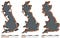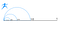From Wikipedia: https://en.wikipedia.org/wiki/How_Long_Is_the_Coast_of_Britain%3F_Statistical_Self-Similarity_and_Fractional_Dimension

# Mandelbrot vs Zeno: Fractals Unravel the Paradox of Motion

There are a few variations of Zeno’s Paradox of Motion and many attempts to resolve it have been proposed. A good summary of the history and solution of this paradox can be found here.

The solution to Zeno’s Paradox has been in the formulation and proof of infinitesimals in calculus. However, if you are looking for a more intuitive explanation that doesn’t invoke calculus then you are in the right place. This solution came to me while investigation Chaos Theory and Fractals.

Like most anything that we find confusing, the quickest way to resolve the issue is to look at the definitions of the concepts used in the argument. Our most obvious target here is distance or length.

The graphic above of Great Britain was inspired by a paper published by Benoit Mandelbrot titled, “How Long Is the Coast of Britain? Statistical Self-Similarity and Fractional Dimension” which was first published in Science in 5 May 1967 (Thank you Wikipedia). This is the same Mandelbrot that coined the term fractal and for whom the famous Mandelbrot Set was named.

There are three geographic images of Great Britain. From left to right a measuring stick of smaller size is used to calculate the length of coastline for the country. As the measuring stick gets smaller accuracy of the measured coastline increases and the total area increases. This is an example of a fractal geometry. You can read more here. The basic consequence is that total length will increase as the measuring stick becomes smaller and smaller.By Miranche — Own work, CC BY-SA 4.0, https://commons.wikimedia.org/w/index.php?curid=55976282

Zeno asserts that any distance can be broken down into an infinite number of small steps. Since it is impossible to complete an infinite number of tasks, motion must be some sort of illusion. It is impossible to do anything infinitely.

However, a big problem with Zeno’s assertion is that the mathematical concept of a straight line and distance is not necessarily the same as the physical concept of distance. You may be able to break down a mathematical line into infinite parts but this may not work the way you’d expect when applied to the physical world. If we consider any surface that Zeno’s race could be held on to have imperfections then it would behave as a fractal geometry. Just like like the length of coastline for Britain increases for smaller and smaller units of measure, each decrease in the units of measure for Achilles would actually make that physical distance longer than when the race started.

The definition of distance does not retain meaning at all scales for all objects. A straight line is an idealized mathematical abstraction of a physical distance. In the real world, a straight line does not truly exist. Zeno’s Paradox asks you to break distances infinitely. Considering that the ground surface is not perfectly smooth, then a fractal geometry would, at some point, require the runner to invoke 2D and even 3D motion to weave in and around the smallest grains of sand or around molecules and atoms to avoid crossing through completely empty or filled space to get from one point to another.

It seems that the way we mathematically define distance is dependent on scale. Breaking down a physical distance infinitely is not reasonable. To me this sounds familiar. This viewpoint may be another perspective of the unresolved boundary that separates classical and quantum mechanics.

I am curious to know what you think. Let me know! Thanks!

Expert Modeler | Scientist | Teacher | Engineer

## More from Jaison Renkenberger

Expert Modeler | Scientist | Teacher | Engineer